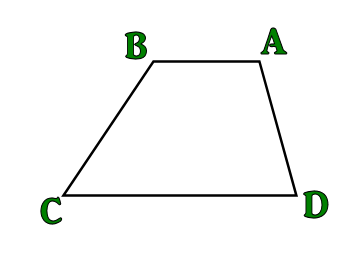Games
Problems
Go Pro!

"example of how to set up trapezoid ABCD, ABllCD, measure of interior angles are distinct and measure ofWell, there are a couple questions in here, one about an angle, and one about how to set up the trapezoid. Here's a picture that shows the setup of the trapezoid. Note that AB and CD are parallel, as the statement of the problem shows. Also note that the letters are in order around the vertices - from A to B to C to D, since the trapezoid is called ABCD. If the trapezoid had been named ACBD, we would have needed to rearrange our vertex names to match that.

We'll come back, in just a second, to the question of how to make sure the interior angle measures are distinct (all different).

But first the question is, how do we find the measure of angle A, if we know that the measure of angle D is x. If you've studied a bit of geometry, you may know that AD is a transversal between parallel lines AB and DC, and that interior angles A and D are consecutive interior angles, which is a fancy way of saying that they're on the same side of the transversal, between the two parallel lines. And consecutive interior angles are supplementary. This means they add to 180 degrees.

Therefore, m

m

m

As for how we make sure the angles are all distinct, there are two things we need to ensure.

First, we need to make sure that none of the angles are 90 degrees, because if ONE of them is 90 degrees, then the associated consecutive interior angle must also be 90 degrees, and that means the angle measures aren't all distinct. Thus, we can't have a right trapezoid.

The other thing is, we need to make sure it's not an isosceles trapezoid (AD = BC). If it's iscoseles, then m

Therefore, if the trapezoid is neither right nor isosceles, the angles will have distinct measures.

# Blogs on This SiteReviews and book lists - books we love!The site administrator fields questions from visitors.Like us on Facebook to get updates about new resources